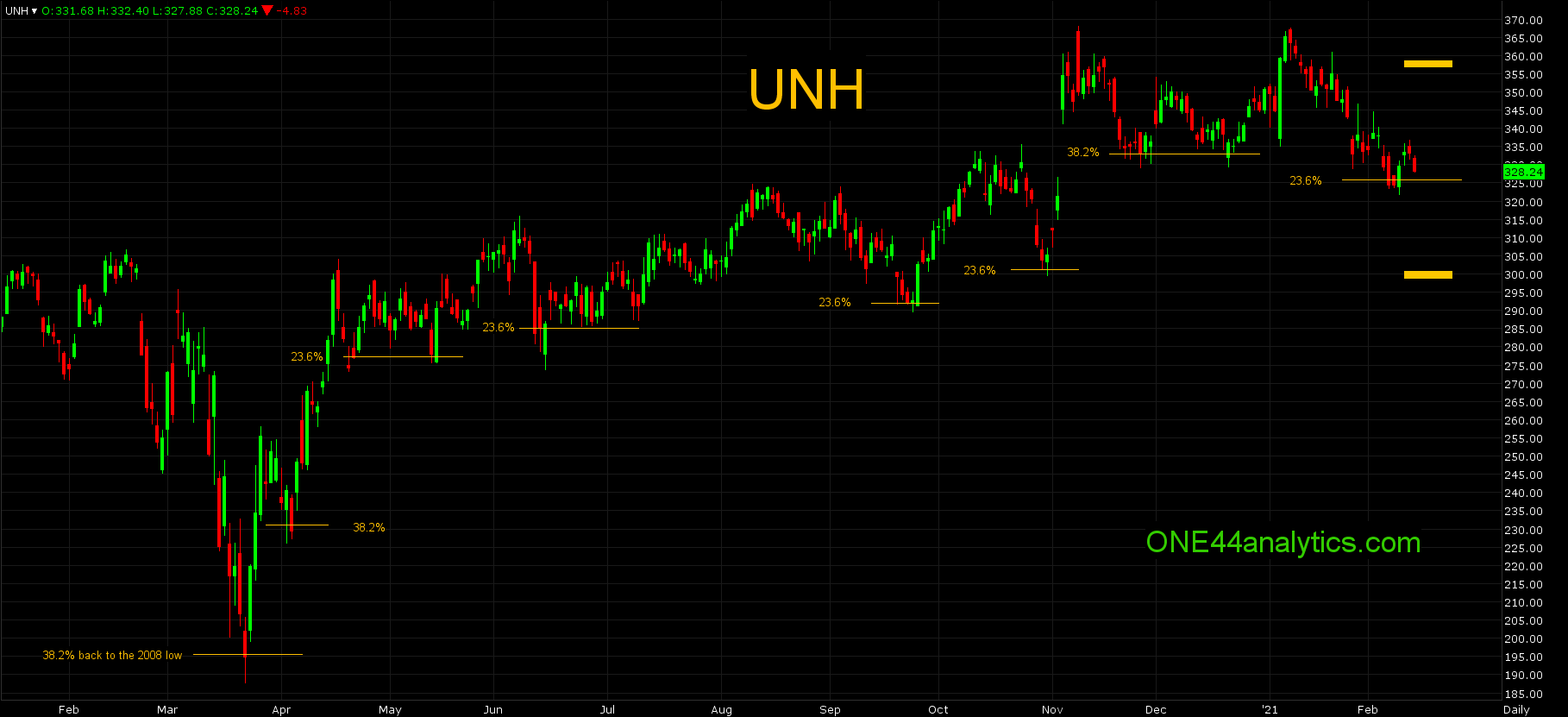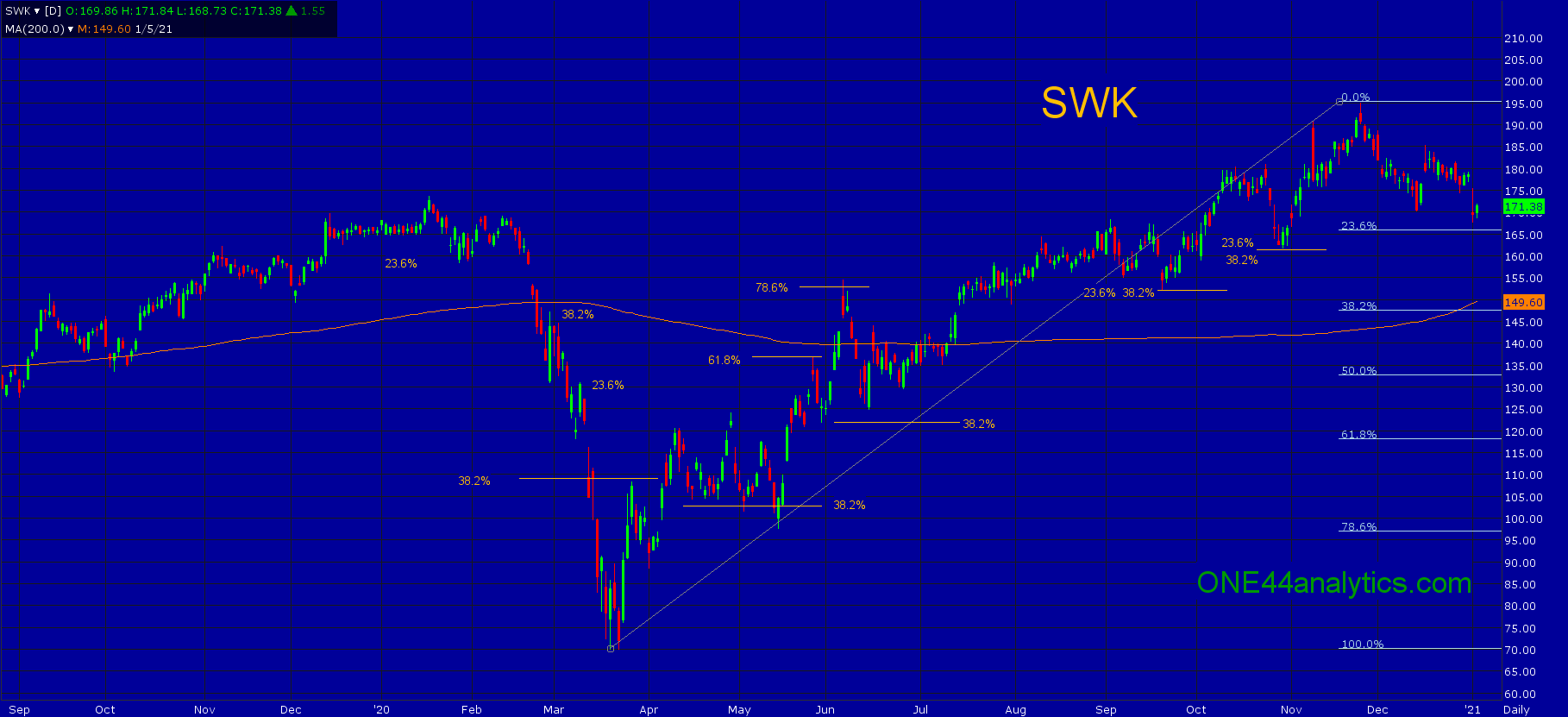# fibonaccichartoftheweek

Aug
02

## Fibonacci chart of the week, Bonds

ONE44 Analytics where the analysis is concise and to the point Our goal is to not only give you actionable
Feb
14## Fibonacci Chart of the Week UNH

ONE44 Analytics where the analysis is concise and to the point In our opinion we believe that the Fibonacci retracements
Feb
05

## S&P

Fibonacci chart of the week ONE44 Analytics where the analysis is concise and to the point S&P 500ESH21
Jan
28

## Fibonacci Chart of the Week

ONE44 Analytics where the analysis is concise and to the point In this example we will go back over a